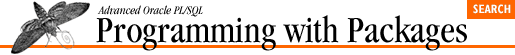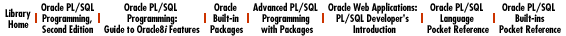home | O'Reilly's CD bookshelfs | FreeBSD | Linux | Cisco | Cisco Exam

### 5.15 PLVio: Input/Output Processing

The PLVio (PL/Vision Input/Output) package consolidates all of the logic required to read from and write to repositories for PL/SQL source code. See Chapter 12, PLVio: Reading and Writing PL/SQL Source Code for details.

### 5.15.1 Package constants and exceptions

``` c_name PLV.plsql_identifier%TYPE := 'name';```
``` c_type PLV.plsql_identifier%TYPE := 'type';```
``` c_text PLV.plsql_identifier%TYPE := 'text';```
``` c_line PLV.plsql_identifier%TYPE := 'line';```
``` c_schema PLV.plsql_identifier%TYPE := 'owner'; ```

The default names for the columns of the database table repository. You can override these names in calls to setsrc and settrg .

``` c_notset CONSTANT VARCHAR2(1) := 'U';```

The value used by PLVio to detect when a repository (source or target) have not yet been set.

``` insert_failure EXCEPTION;```

Exception raised when PLVio is unable to insert or put a line to the target repository.

### 5.15.2 Package records

``` TYPE line_type IS RECORD```
``` (text VARCHAR2(2000) := NULL,```
``` len INTEGER := NULL,```
``` pos INTEGER := 1,```
``` line INTEGER := 0, /* line # in original */```
``` line# INTEGER := 0, /* line # for new */```
``` is_blank BOOLEAN := FALSE,```
``` eof BOOLEAN := FALSE,```
``` indent INTEGER := 0,```
``` unindent BOOLEAN := FALSE,```
``` continuation BOOLEAN := FALSE,```
``` blank_line_before BOOLEAN := FALSE);```

The line_type record TYPE defines the structure for a line datatype in PLVio.

``` empty_line line_type;```

Predefined empty line that can be used to initialize a line's fields.

### 5.15.3 Source and target repository type functions

``` FUNCTION file_source RETURN BOOLEAN;```
``` FUNCTION pstab_source RETURN BOOLEAN;```
``` FUNCTION dbtab_source RETURN BOOLEAN;```
``` FUNCTION string_source RETURN BOOLEAN;```

Returns TRUE if the current source repository matches that indicated by the name, FALSE otherwise.

``` FUNCTION file_target RETURN BOOLEAN;```
``` FUNCTION pstab_target RETURN BOOLEAN;```
``` FUNCTION dbtab_target RETURN BOOLEAN;```
``` FUNCTION string_target RETURN BOOLEAN;```
``` FUNCTION stdout_target RETURN BOOLEAN;```

Returns TRUE if the current target repository matches that indicated by the name, FALSE otherwise.

``` FUNCTION nosrc RETURN BOOLEAN;```
``` FUNCTION notrg RETURN BOOLEAN;```

These functions return TRUE if the source and target repositories, respectively, have not yet been set.

``` FUNCTION srctype RETURN VARCHAR2;```
``` FUNCTION trgtype RETURN VARCHAR2; ```

These functions return the current source and target repository types. The values returned can be matched against constants in the PLV package.

### 5.15.4 Managing the source repository

``` PROCEDURE setsrc```
``` (srctype_in IN VARCHAR2, ```
``` name_in IN VARCHAR2 := 'user_source',```
``` name_col_in IN VARCHAR2 := c_name,```
``` type_col_in IN VARCHAR2 := c_type,```
``` line#_col_in IN VARCHAR2 := c_line,```
``` text_col_in IN VARCHAR2 := c_text,```
``` schema_col_in IN VARCHAR2 := NULL);```

This procedure sets the source repository. You provide the repository type, the name, and then, if a database table, the names of the columns in the table.

``` PROCEDURE initsrc```
``` (starting_at_in IN INTEGER,```
``` ending_at_in IN INTEGER,```
``` where_in IN VARCHAR2 := NULL);```
``` PROCEDURE initsrc```
``` (starting_at_in IN VARCHAR2 := NULL,```
``` ending_at_in IN VARCHAR2 := NULL,```
``` where_in IN VARCHAR2 := NULL);```

The initsrc procedures initialize the source after it has been set. You can provide additional information in the call to initsrc to restrict which rows are retrieved from the source, including a WHERE clause and start-end line numbers or strings.

``` PROCEDURE usrc```
``` (starting_at_in IN VARCHAR2 := NULL,```
``` ending_at_in IN VARCHAR2 := NULL,```
``` where_in IN VARCHAR2 := NULL);```
``` PROCEDURE usrc```
``` (starting_at_in IN INTEGER,```
``` ending_at_in IN INTEGER,```
``` where_in IN VARCHAR2 := NULL);```

The two usrc procedures set the source repository to the USER_SOURCE data dictionary view and then initialize the source with a call to initsrc , passing along the arguments provided to it (notice that they match those of initsrc ).

``` PROCEDURE asrc```
``` (starting_at_in IN VARCHAR2 := NULL,```
``` ending_at_in IN VARCHAR2 := NULL,```
``` where_in IN VARCHAR2 := NULL);```
``` PROCEDURE asrc ```
``` (starting_at_in IN INTEGER,```
``` ending_at_in IN INTEGER,```
``` where_in IN VARCHAR2 := NULL);```

The two asrc procedures set the source repository to the ALL_SOURCE data dictionary view and then initialize the source with a call to initsrc , passing along the arguments provided to it (notice that they match those of initsrc ).

``` FUNCTION srcselect RETURN VARCHAR2;```

Returns the current SELECT statement associated with the source repository. This is only relevant when the source type is a database table ( PLV.dbtab ).

``` PROCEDURE closesrc;```

Closes the source repository. If a database table, the cursor is closed. If a file, the file is closed.

### 5.15.5 Managing the source WHERE clause

When the source type is a database table, you can manipulate the WHERE clause which identifies or restricts those rows that are read from the source table. The following constants and programs all have an impact on the WHERE clause.

``` c_first CONSTANT VARCHAR2(1) := 'F';```
``` c_last CONSTANT VARCHAR2(1) := 'L';```
``` c_before CONSTANT VARCHAR2(1) := 'B';```
``` c_after CONSTANT VARCHAR2(1) := 'A';```

These constants indicate the type of match to be performed when using the line_with and set_line_limit procedures.

``` PROCEDURE set_srcwhere (where_in IN VARCHAR2 := NULL);```

Sets the source repository WHERE clause, either by replacing it or providing additional elements.

``` PROCEDURE rem_srcwhere;```

Removes any additional elements that have been added to the WHERE clause.

``` FUNCTION line_with ```
``` (text_in IN VARCHAR2,```
``` loc_type_in IN VARCHAR2 := c_first,```
``` after_in IN INTEGER := NULL)```
``` RETURN INTEGER;```
``` FUNCTION line_with ```
``` (text_in IN VARCHAR2,```
``` loc_type_in IN VARCHAR2 := c_first,```
``` after_in IN VARCHAR2,```
``` after_loc_type_in IN VARCHAR2 := c_first)```
``` RETURN INTEGER;```

The line_with functions return the line number associated with the values passed to them. They answer questions like "What is the first line in the PLVvu package containing `IF'?" and "What is the last line in the PLV package containing `CONSTANT' that comes after the string `VARCHAR2'?"

``` PROCEDURE set_line_limit```
``` (line_in IN INTEGER, loc_type_in IN VARCHAR2 := c_first);```

Adds an element to the WHERE clause of the source repository restricting the text retrieved by a line number.

``` PROCEDURE rem_line_limit (line_in IN INTEGER);```

Use this procedure to remove from the WHERE clause an element added by set_line_limit .

### 5.15.6 Managing the target repository

``` target_table PLVtab.vc2000_table;```

The PL/SQL table that contains the code when the target is set to PL/SQL table.

``` target_row BINARY_INTEGER;```

The number of rows in the target PL/SQL table.

``` PROCEDURE settrg```
``` (trgtype_in IN VARCHAR2,```
``` name_in IN VARCHAR2 := 'PLV_source',```
``` target_name_col_in IN VARCHAR2 := 'name',```
``` trgtype_col_in IN VARCHAR2 := 'type',```
``` target_line#_col_in IN VARCHAR2 := 'line',```
``` target_text_col_in IN VARCHAR2 := 'text');```

Sets the target and, if a database table, the structure of the table containing the text. This program also calls inittrg (not so with the source repository).

``` PROCEDURE disptrg```
``` (header_in IN VARCHAR2 := NULL,```
``` start_in IN INTEGER := 1,```
``` end_in IN INTEGER := target_row,```
``` type_in IN VARCHAR2 := trgtype);```

Displays the contents of the target repository. The second and third arguments apply to PL/SQL tables and file repositories only. The fourth argument allows you to override the current target type to display the contents of another type repository.

``` PROCEDURE inittrg;```

Initializes the target repository; this is called by settrg so there is very little reason to execute this directly.

``` FUNCTION trgstg RETURN VARCHAR2;```

Returns the string target repository. Separate lines of text in the repository are separated by a CHR(10) character.

``` PROCEDURE closetrg;```

Closes the target repository.

``` PROCEDURE clrtrg ```
``` (program_name_in IN VARCHAR2 := NULL,```
``` program_type_in IN VARCHAR2 := NULL);```

Clears the specified repository. If a database table, the rows are deleted. If a string or PL/SQL table, the repository is set to NULL.

### 5.15.7 Reading and writing lines

The whole point of PLVio is to read from the source repository and/or write to the target repository. In PLVio lingo, this means that you get a line from the source and put a line to the target.

``` PROCEDURE initline```
``` (line_inout IN OUT line_type,```
``` text_in IN VARCHAR2 := NULL,```
``` len_in IN INTEGER := NULL,```
``` pos_in IN INTEGER := 1,```
``` line#_in IN INTEGER := 0,```
``` is_blank_in IN BOOLEAN := FALSE,```
``` eof_in IN BOOLEAN := FALSE);```

Initializes a line record (defined with the PLV io.line_type record TYPE) with the values provided in the parameter list.

``` PROCEDURE get_line ```
``` (line_inout IN OUT line_type,```
``` curr_line#_in IN INTEGER := NULL);```

Gets a line from the source repository and deposits it in the line record provided in the argument list.

``` FUNCTION rest_of_line```
``` (line_in IN line_type, pos_in IN INTEGER := line_in.pos) ```
``` RETURN VARCHAR2;```

Returns the rest of the line that has not yet been scanned, based on the current position in the line (provided by the second argument).

``` PROCEDURE put_line (line_in IN line_type);```

Puts a line record in the target repository.

``` PROCEDURE put_line```
``` (string_in IN VARCHAR2, line#_in IN INTEGER := NULL);```

Puts a string in the target repository. Use this version of put_line when you are not otherwise working with a record defined with the line_type record TYPE and simply have a string to move to the repository.

### 5.15.8 Saving and restoring repository settings

``` PROCEDURE savesrc;```

Requests that the current settings for the source repository be saved and then restored upon close of the (new) source (the default).

``` PROCEDURE nosavesrc;```

Requests that saves and restores not be performed.

``` FUNCTION saving_src RETURN BOOLEAN;```

Returns TRUE if saves and restores are being performed.

``` PROCEDURE savetrg;```

Requests that the current settings for the target repository be saved and then restored upon close of the (new) target (the default).

``` PROCEDURE nosavetrg;```

Requests that saves and restores for the target not be performed.

``` FUNCTION saving_trg RETURN BOOLEAN;```

Returns TRUE if saves and restores for the target are being performed.

``` PROCEDURE restoresrc;```

Restores the source repository to its previous value.

``` PROCEDURE restoretrg;```

Restores the target repository to its previous value.

### 5.15.9 Miscellaneous PLVio programs

``` PROCEDURE src2trg (close_in IN BOOLEAN := TRUE);```

Transfers the contents of the source repository directly to the target repository.

### 5.15.10 Tracing PLVio activity

``` PROCEDURE display;```

Requests that PLVio actions be displayed as they occur.

``` PROCEDURE nodisplay;```

Requests that PLVio actions not be displayed as they occur.

``` FUNCTION displaying RETURN BOOLEAN;```

Returns TRUE if PLVio is displaying its actions.5.14 PLVhlp: Online Help Architechture5.16 PLVlex: Lexical Analysis